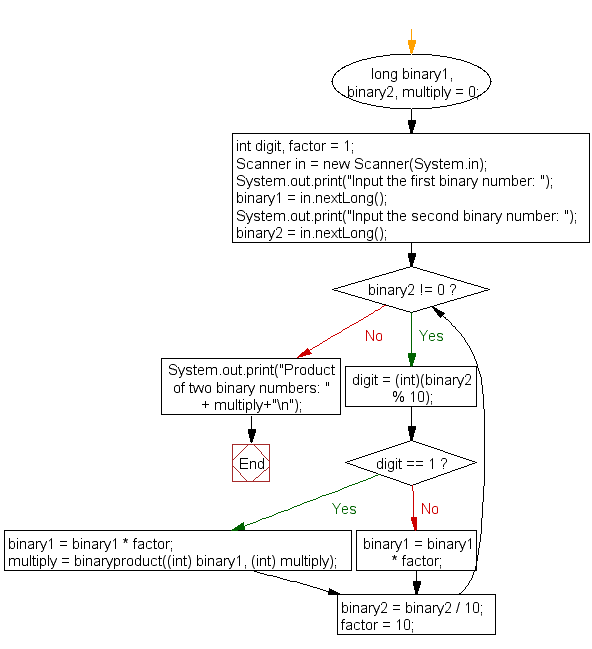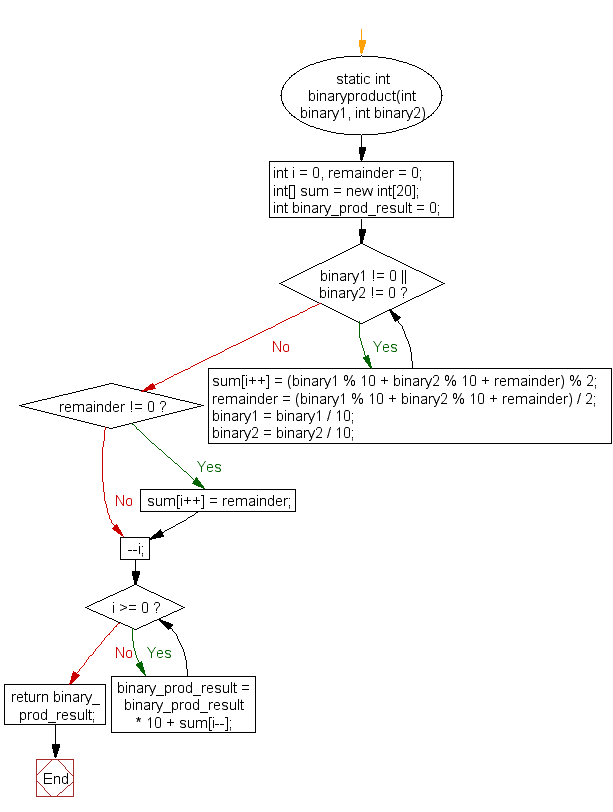﻿ Java exercises: Multiply two binary numbers - w3resource# Java Exercises: Multiply two binary numbers

## Java Basic: Exercise-18 with Solution

Write a Java program to multiply two binary numbers.

In digital electronics and mathematics, a binary number is a number expressed in the base-2 numeral system or binary numeral system. This system uses only two symbols: typically 1 (one) and 0 (zero).

Test Data:
Input first binary number: 110
Input second binary number: 101

Sample Solution:

Java Code:

``````import java.util.Scanner;
public class Exercise18 {
public static void main(String[] args)
{
long binary1, binary2, multiply = 0;
int digit, factor = 1;
Scanner in = new Scanner(System.in);
System.out.print("Input the first binary number: ");
binary1 = in.nextLong();
System.out.print("Input the second binary number: ");
binary2 = in.nextLong();
while (binary2 != 0)
{
digit = (int)(binary2 % 10);
if (digit == 1)
{
binary1 = binary1 * factor;
multiply = binaryproduct((int) binary1, (int) multiply);
}
else
{
binary1 = binary1 * factor;
}
binary2 = binary2 / 10;
factor = 10;
}
System.out.print("Product of two binary numbers: " + multiply+"\n");
}
static int binaryproduct(int binary1, int binary2)
{
int i = 0, remainder = 0;
int[] sum = new int;
int binary_prod_result = 0;

while (binary1 != 0 || binary2 != 0)
{
sum[i++] = (binary1 % 10 + binary2 % 10 + remainder) % 2;
remainder = (binary1 % 10 + binary2 % 10 + remainder) / 2;
binary1 = binary1 / 10;
binary2 = binary2 / 10;
}
if (remainder != 0)
{
sum[i++] = remainder;
}
--i;
while (i >= 0)
{
binary_prod_result = binary_prod_result * 10 + sum[i--];
}
return binary_prod_result;
}
}
```
```

Sample Output:

```Input the first binary number: 110
Input the second binary number: 101
Product of two binary numbers: 11110
```

Flowchart:Flowchart : binaryproduct()Java Code Editor:

What is the difficulty level of this exercise?

Test your Programming skills with w3resource's quiz.

﻿

## Java: Tips of the Day

getEnumMap

Converts to enum to Map where key is the name and value is Enum itself.

```public static <E extends Enum<E>> Map<String, E> getEnumMap(final Class<E> enumClass) {
return Arrays.stream(enumClass.getEnumConstants())
.collect(Collectors.toMap(Enum::name, Function.identity()));
}
```

Ref: https://bit.ly/3xXcFZt